• 关键为表达式求值函数的编写 对该函数，循环结束条件为栈顶元素为’#’,且读入的字符也为’#’ 循环体 （1）判断是否为操作数，是操作数，则压入栈中读取下一个字符 （2）是操作符,则比较操作符的优先级 操作符...

实验原理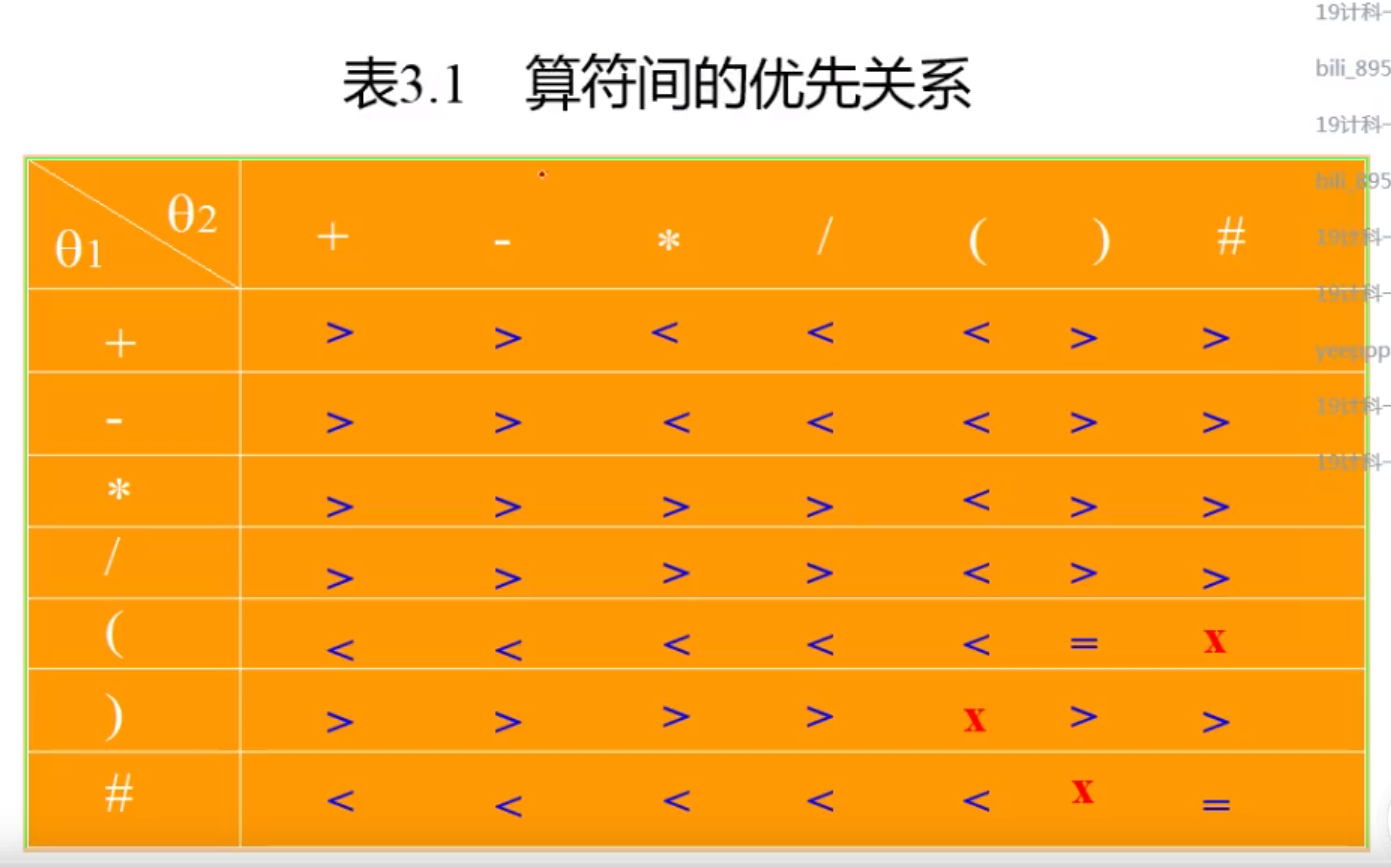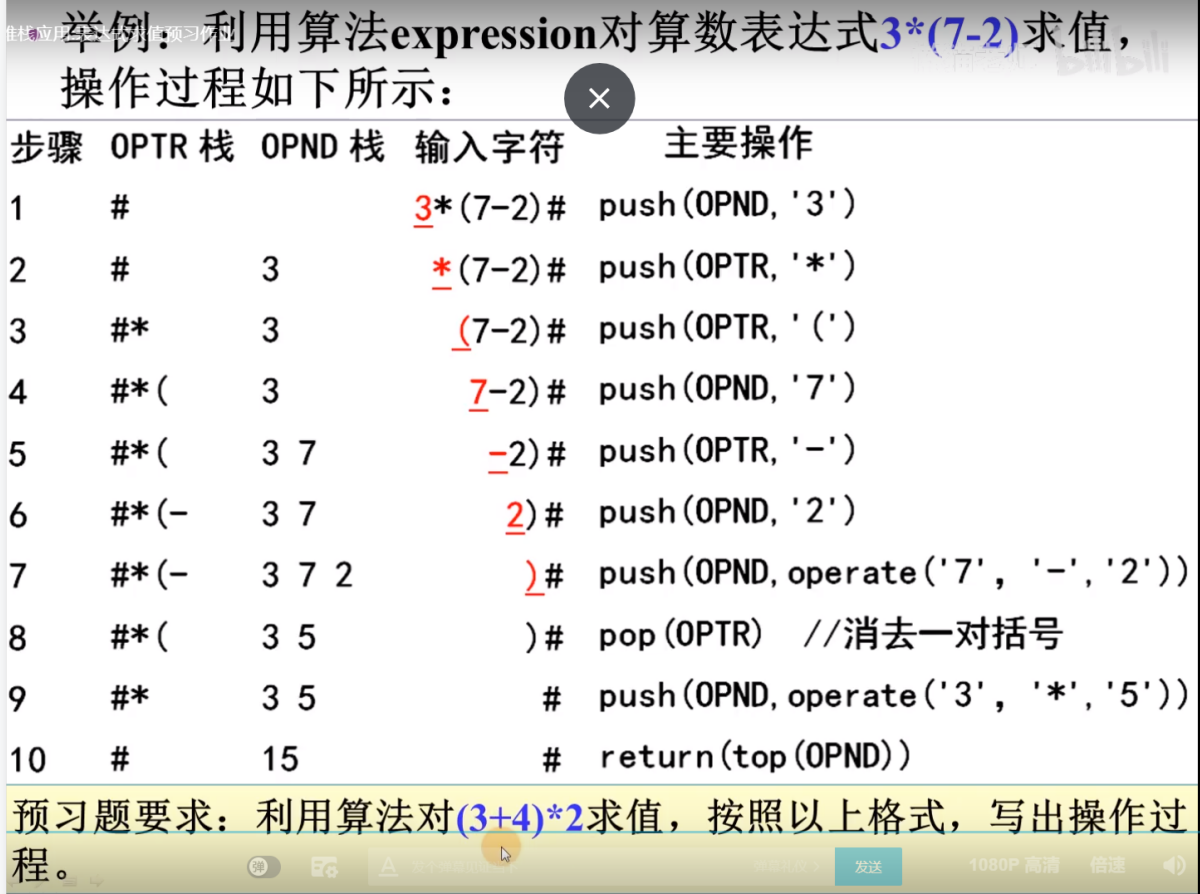代码思路

• 使用两个顺序栈编程，一个顺序栈用来存储操作数，一个顺序栈用来存储操作符

• 关键为表达式求值函数的编写

• 对该函数，循环结束条件为栈顶元素为’#’,且读入的字符也为’#’

• 循环体

• （1）判断是否为操作数，是操作数，则压入栈中读取下一个字符
• （2）是操作符,则比较操作符的优先级
• 操作符栈栈顶元素用t1表示，读入的字符用t2表示
• （a)t1>t2,则t1弹出操作符栈，与此同时操作数栈弹出两个元素，进行运算操作，将结果压入操作数栈中，注意该操作不读取下一个字符
• (b)t1 = t2,操作符栈弹出栈顶元素，读取下一个字符
• 接下来便是precede函数，isOptr函数，operate函数的编写

实验代码（采用C++面向对象编程）

stack.h

#ifndef STACK_H__
#define STACK_H__
#define MAXSIZE 20
template<class DataType>
class Stack
{
private:
DataType *data;
int top;
int capacity;
public:
Stack();//无参构造函数
Stack(int sz);//有参构造函数
~Stack();//析构函数
DataType Pop();//弹出元素
void Push(DataType elem);//压入元素
DataType getTop();//得到栈顶元素
bool isEmpty();//判断栈是否为空
bool isFull();//判断栈是否为满
class Empty{};//设置内置异常类
class Full {};
};
typedef Stack<char> CharStack;
typedef Stack<int> IntStack;
int evaluatExpression(IntStack &opndStack, CharStack &optrStack);
#endif

stack.cpp

#include<iostream>
using namespace std;
#include "stack.h"

template<class DataType>
Stack<DataType>::Stack()
{
data = new DataType[MAXSIZE];
top = -1;
capacity = MAXSIZE;
}

template<class DataType>
Stack<DataType>::Stack(int sz)
{
data = new DataType[sz];
top = -1;
capacity = sz;
}

template<class DataType>
Stack<DataType>::~Stack()
{
delete[]  data;
}

template<class DataType>
DataType Stack<DataType>::Pop()
{
if (isEmpty())
{
throw Empty();
}
else
{
return data[top--];
}
}

template<class DataType>
void  Stack<DataType>::Push(DataType elem)
{
if (isFull())
{
throw Full();
}
else
{
data[++top] = elem;
}
}

template<class DataType>
DataType Stack<DataType>::getTop()
{
if (isEmpty())
{
throw Empty();
}
else
{
return data[top];
}
}

template<class DataType>
bool Stack<DataType>::isEmpty()
{
if (top == -1)
{
return true;
}
else
{
return false;
}
}

template<class DataType>
bool Stack<DataType>::isFull()
{
if (top == MAXSIZE - 1)
{
return true;
}
else
{
return false;
}
}

template class Stack<char>;
template class Stack<int>;

int operate(int num1,char optr, int num2)
{
switch (optr)
{
case '+':
return num1 + num2;
break;
case '-':
return num2 - num1;
break;
case '*':
return num2 * num1;
break;
case '/':
return num2 / num1;
break;
}
}

bool isOptr(char ch)
{
switch (ch)
{
case '+':
return true;
break;
case '-':
return true;
break;
case '*':
return true;
break;
case '/':
return true;
break;
case '(':
return true;
break;
case ')':
return true;
break;
case '#':
return true;
break;
default:
return false;
break;
}
}
//判断操作符的优先级,t1优先级高于t2优先级返回'>'
char  precede(char t1, char t2)
{
char f;
switch (t1)
{
case '+':
if (t2 == '*' || t2 == '/' || t2 == '(')
{
f = '<';
}
else
{
f = '>';
}
break;
case '-':
if (t2 == '*' || t2 == '/' || t2 == '(')
{
f = '<';
}
else
{
f = '>';
}
break;
case '*':
if (t2 == '(')
{
f = '<';
}
else
{
f = '>';
}
break;
case '/':
if (t2 == '(')
{
f = '<';
}
else
{
f = '>';
}
break;
case '(':
if (t2 == ')')
{

f = '=';
}
else if (t2 == '#')
{
f = ' ';
}
else
{
f = '<';
}
break;
case ')':
if (t2 == '(')
{
f = ' ';
}
else
{
f = '>';
}
break;
case '#':
if (t2 == '#')
{
f = '=';
}
if (t2 == ')')
{
f = ' ';
}
else
{
f = '<';
}
break;
}
return f;
}
int evaluatExpression(IntStack &opndStack, CharStack &optrStack)
{
char ch,optr;
int num1, num2,res,temp;
optrStack.Push('#');
int isNum = 0;//判断前一个字符是否为数字
cin >> ch;
//循环结束条件,栈顶元素为'#',且读入的字符为'#'
while (ch != '#' || optrStack.getTop() != '#')
{
if (!isOptr(ch))//操作数,压入数据栈中
{
if (isNum == 1)
{
temp = opndStack.Pop();
temp = temp * 10 + (ch - '0');
opndStack.Push(temp);
isNum = 1;
cin >> ch;
}
else
{
isNum = 1;
opndStack.Push(ch - '0');
cin >> ch;
}

}
else//操作符，比较优先级
{
isNum = 0;
switch (precede(optrStack.getTop(),ch))
{
case '>'://栈顶元素出栈，操作数栈弹出2个元素
num1 = opndStack.Pop();
num2 = opndStack.Pop();
/*cout << num1 << "\t";
cout << num2 << endl;*/
optr = optrStack.Pop();
res = operate(num1, optr, num2);
opndStack.Push(res);
break;
case '<':
optrStack.Push(ch);
cin >> ch;
break;
case '=':
optrStack.Pop();
cin >> ch;
break;
}
}
}
return opndStack.Pop();
}

main.c

#include<iostream>
using namespace std;
#include "stack.h"
//表达式求值
int main()
{
CharStack optrStack;
IntStack opndStack;
NELEMTYPE res;
cout << "请输入算数表达式以#结束" << endl;
res = EvaluateExpression(optrStack, opndStack);
cout << res << endl;

return 0;
}

运行结果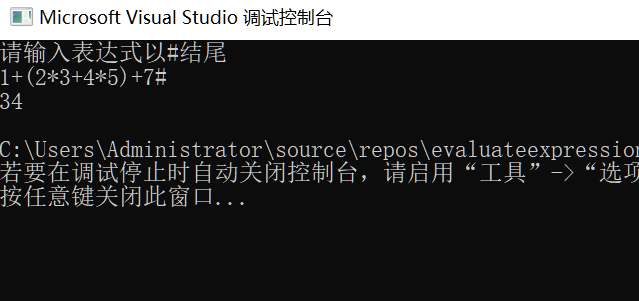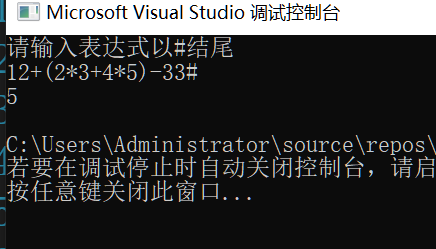参考链接

b站up主：laura柳b站up主:跟懒猫老师快乐数据结构展开全文c++ 数据结构 算法
• 数据结构表达式求值(计算器)实验报告(共10篇)数据结构表达式求值(计算器)实验报告(共10篇)数据结构课程设计_实验报告(一)表达式求值(计算器)数据结构课程设计 实验报告起止时间：2015.12.28-2015.12.31附件：(程序源...

数据结构表达式求值(计算器)实验报告(共10篇)

数据结构表达式求值(计算器)实验报告(共10篇)

数据结构课程设计_实验报告(一)表达式求值(计算器)

数据结构课程设计 实验报告

起止时间：2015.12.28-2015.12.31

附件：(程序源代码)

#includestdio.h

#includestdlib.h

#includestring.h

#includemath.h

#define N 100

#define pai 3.1415926

typedef struct yxj

{

char operat;

int rank;

}yxj;

typedef struct str

{

char data[N];

}zs;

void sjhs(void)

{

char s,a;

double y,x;

printf(请输入(sin cos tan 角度制)表达式：\n);

scanf(%s,s);

if(strstr(s(来自:www.XIelw.Com 写 论文网:),sin)!=0)

{

int i=0,j=0;

while(s[i]!='\0')

{

if(s[i]='0'&&s[i]='9')

s[j++]=s[i];

i++;

}

s[j]='\0';

x=atof(s);

y=sin(x*pai/180);

}

else if(strstr(s,cos)!=0)

{

int i=0,j=0;

while(s[i]!='\0')

{

if(s[i]='0'&&s[i]='9')

s[j++]=s[i];

i++;

}

篇二：数据结构算术表达式求值实验报告

软件技术基础实验报告

实验名称： 系 别： 年 级：

班 级： 学生学号： 学生姓名：

表达式计算器

通信工程 2012

2 23320122203902 李 斐 1

《数据结构》课程设计报告

题目 简易计算表达式的演示

【题目要求】

要求：实现基本表达式计算的功能

输入：数学表达式，表达式由整数和“+”、 “-”、“×”、“/”、“(”、“)”组成 输出：表达式的值

基本操作：键入表达式，开始计算，计算过程和结果记录在文档中 难点：括号的处理、乘除的优先级高于加减

1．前言

在计算机中，算术表达式由常量、变量、运算符和括号组成。由于不同的运算符具有不同的优先级，又要考虑括号，因此，算术表达式的求值不可能严格地从左到右进行。因而在程序设计时，借助栈实现。

算法输入：一个算术表达式，由常量、变量、运算符和括号组成(以字符串形式输入)。为简化，规定操作数只能为正整数，操作符为+、-*、/、=，用#表示结束。

算法输出：表达式运算结果。

算法要点：设置运算符栈和运算数栈辅助分析算符优先关系。在读入表达式的字符序列

2

的同时，完成运算符和运算数的识别处理，以及相应运算。

2．概要设计

2.1 数据结构设计

任何一个表达式都是由操作符，运算符和界限符组成的。我们分别用顺序栈来寄存表达式的操作数和运算符。栈是限定于紧仅在表尾进行插入或删除操作的线性表。顺序栈的存储结构是利用一组连续的存储单元依次存放自栈底到栈顶的数据元素，同时附设指针top指示栈顶元素在顺序栈中的位置，base为栈底指针，在顺序栈中，它始终指向栈底，即top=base可作为栈空的标记，每当插入新的栈顶元素时，指针top增1，删除栈顶元素时，指针top减1。

2.2 算法设计

为了实现算符优先算法。可以使用两个工作栈。一个称为OPTR，用以寄存运算符，另一个称做OPND，用以寄存操作数或运算结果。

1.首先置操作数栈为空栈，表达式起始符”#”为运算符栈的栈底元素；

2.依次读入表达式，若是操作符即进OPND栈，若是运算符则和OPTR栈的栈顶运算符比较优先权后作相应的操作，直至整个表达式求值完毕(即OPTR栈的栈顶元素和当前读入的字符均为”#”)。

数据对象：D={ai |ai∈ElemSet,i=1,2,?，n, n≧0} 数据对象：R1={ai,ai?1|ai?1,ai?D,i=2,?，n} 约定an端为栈顶，ai端为栈底。 基本操作：

InitStack(&S)

操作结果：构造一个空栈S。GetTop(S)

初始条件：栈S已存在。

3

操作结果：用P返回S的栈顶元素。Push(&S，ch) 初始条件：栈S已存在。

操作结果：插入元素ch为

展开全文• //计算表达式值 void OPTR_InitStack(SqStack1 *S); //运算符栈的初始化 void OPND_InitStack(SqStack2 *S); //操作数栈的初始化 char OPTR_GetTop(SqStack1 S); //取运算符栈的栈顶元素 int OPND_GetTop(SqStack2 ...

该楼层疑似违规已被系统折叠 隐藏此楼查看此楼

#include

#include

#include

#define STACK_INIT_SIZE 100 //存储空间初始分配量

#define STACKINCREMENT 10 //存储空间分配增量

#define OK 1

#define ERROR 0

typedef struct{

char *base;

char *top;

int stacksize;

}SqStack1; //运算符栈类型定义

typedef struct{

int *base;

int *top;

int stacksize;

}SqStack2; //操作数栈类型定义

SqStack1 OPTR; //运算符栈

SqStack2 OPND; //操作数栈

int EvaluateExpression(); //计算表达式值

void OPTR_InitStack(SqStack1 *S); //运算符栈的初始化

void OPND_InitStack(SqStack2 *S); //操作数栈的初始化

char OPTR_GetTop(SqStack1 S); //取运算符栈的栈顶元素

int OPND_GetTop(SqStack2 S); //取操作数栈的栈顶元素

char OPTR_Push(SqStack1 *S, char e); //压栈

int OPND_Push(SqStack2 *S, int e); //压栈

char OPTR_Pop(SqStack1 *S, char *e); //出栈

int OPND_Pop(SqStack2 *S, int *e); //出栈

char Precede(char a, char b); //运算符优先级比较

int Operate( int d, char e,int f); //操作数d、f参加e运算的结果

int In(char c); //判断c是否是运算符

void OPTR_Init_Stack(SqStack1 *S)//运算符栈的初始化

{

(*S).base=(char *)malloc(STACK_INIT_SIZE*sizeof(char));

if(!(*S).base) exit (OVERFLOW);//存储分配失败

(*S).top=(*S).base;

(*S).stacksize=STACK_INIT_SIZE;

}

void OPND_Init_Stack(SqStack2 *S)

{

(*S).base=(int *)malloc(STACK_INIT_SIZE*sizeof(int));

if(!(*S).base) exit (OVERFLOW);//存储分配失败

(*S).top=(*S).base;

(*S).stacksize=STACK_INIT_SIZE;

}

char OPTR_GetTop(SqStack1 S)//取运算符栈的栈顶元素

{

if(S.top==S.base) return ERROR;

return *(S.top-1);

return OK;

}

int OPND_GetTop(SqStack2 S) //取操作数栈的栈顶元素

{

if(S.top==S.base) return ERROR;

return *(S.top-1);

return OK;

}

char OPTR_Push(SqStack1 *S, char e) //压栈

{

if ((*S).top-(*S).base>=(*S).stacksize)

{

(*S).base=(char *)realloc

((*S).base,((*S).stacksize+STACKINCREMENT)*sizeof(char));

if(!(*S).base) exit(ERROR);

(*S).top=(*S).base+(*S).stacksize;

(*S).stacksize+=STACKINCREMENT;

}

*(*S).top=e;

(*S).top++;

return OK;

}

int OPND_Push(SqStack2 *S, int e) //压栈

{

if ((*S).top-(*S).base>=(*S).stacksize)

{

(*S).base=(int *)realloc

((*S).base,((*S).stacksize+STACKINCREMENT)*sizeof(int));

if(!(*S).base) exit(ERROR);

(*S).top=(*S).base+(*S).stacksize;

(*S).stacksize+=STACKINCREMENT;

}

*(*S).top=e;

(*S).top++;

return OK;

}

char OPTR_Pop(SqStack1 *S, char *e) //出栈

{

if((*S).top==(*S).base) return ERROR;

*e=*--(*S).top;

return OK;

}

int OPND_Pop(SqStack2 *S, int *e) //出栈

{

if((*S).top==(*S).base) return ERROR;

*e=*--(*S).top;

return OK;

}

char Precede(char a, char b) //运算符优先级比较

{

char Op;

switch(a)

{

case '#':if(b=='#')

Op='=';

else Op='

case '+':if(b=='*' || b=='/' || b=='(' || b=='^')

Op='

else Op='>';break;

case '*':if(b=='(' || b=='^' )

Op='

else Op='>';break;

case '/':if(b=='(' || b=='^')

Op='

else Op='>';break;

case '(':if(b==')')

Op='=';

else Op='

case ')':Op='>';break;

case '^':if(b=='(')

Op='

else Op='>'; break;

}

return Op;

}

int Operate( int d, char e,int f) //操作数d、f参加e运算的结果

{

int g;

switch(e)

{

case '+':g=d+f;break;

case '-':g=d-f;break;

case '*':g=d*f;break;

case '/':g=d/f;break;

}

return g;

}

int In(char c) //判断c是否是运算符

{

if(c=='#' || c=='(' || c==')' || c=='+' || c=='-' || c=='*' || c=='/' || c=='^') return OK;

else if(c>='0' && c<='9') return OK;

else return ERROR;

}

int EvaluateExpression() //计算表达式值

{

int a,b;

char c,theta,x;

printf("请输入表达式\n");

OPTR_Init_Stack(&OPTR);OPTR_Push(&OPTR,'#');

OPND_Init_Stack(&OPND);c=getchar();

while(c!='#'||OPTR_GetTop(OPTR)!='#'){

if(!In(c))

{

OPND_Push(&OPND,(c-'0'));c=getchar();//不是运算符则进栈

}

else

switch(Precede(OPTR_GetTop(OPTR),c)){

case'

OPTR_Push(&OPTR,c);c=getchar();

break;

case'='://脱括号并接收下一字符

OPTR_Pop(&OPTR,&x);c=getchar();

break;

case'>'://退栈并将运算结果入栈

OPTR_Pop(&OPTR,&theta);

OPND_Pop(&OPND,&b);OPND_Pop(&OPND,&a);

OPND_Push(&OPND,Operate(a,theta,b));

break;

}

}

return OPND_GetTop(OPND);

}

int main()

{

int result;

result = EvaluateExpression();

printf("%d\n", result);

return OK;

}

展开全文c语言表达式的值为0
• 下面是用栈实现表达式求值的一个实例，用的是顺序栈的形式 原理： List item 我们默认一‘#’开始，最后输入’#‘结束 在运算中的每一步中，任意两个相继出现的算符theta1和theta2之间的关系，至多只有三种关系： ...

最近学习c语言的数据结构中有关栈的实现。下面是用栈实现表达式求值的一个实例，用的是顺序栈的形式
原理

1. List item

我们默认一‘#’开始，最后输入’#‘结束

在运算中的每一步中，任意两个相继出现的算符theta1和theta2之间的关系，至多只有三种关系：
1,theta1<theta2
2,theta1>theta2
3,theta1=theta2
theta1和theta2之间的关系由下表给出根据算数运算规则的左结合性，先压入栈的同型号运算符优先级要大些，比如栈顶为‘+’，输入为‘+’，此时该算那个‘+’呢，应该算栈顶那个‘+’；
因为我们无法比较（和#，（，#，）之间的优先级关系所以如果输入这种运算符的话程序将会出错，所以在程序中应注意避免
算法
该算法把数字和字符都以char型入栈
1）初始化opnd（数字）和optr（字符）栈，将#压入oper栈
2）循环表达式如果输入的值ch非#和栈顶元素非#则执行以下操作：
若输入的值不是运算符，则压入opnd（数字）栈
否则将输入的值与栈顶进行比较
若是<则ch压入optr栈，读入下一字符
若是>则optr弹出运算符，opnd弹出两个元素，和运算符进行运算
若是=则optr栈顶元素是(且ch是），弹出optr栈顶，相当于括号匹配成功
3）最后循环结束opnd栈顶元素即为所求值
以下是源代码，所用软件是visualstudio2022

#include<stdio.h>
#include<stdlib.h>
#include<malloc.h>
#include"pch.h"

#define STACK_CHAR_SIZE 100
#define STACKINCREMENT 10

typedef struct {

char* base;
char* top;
int stacksize;
}SqStack;

//获取theta所对应的索引
//返回索引值
int getIndex(char theta)
{
int index = -1;
switch (theta)
{
case '+':
index = 0;
break;
case '-':
index = 1;
break;
case '*':
index = 2;
break;
case '/':
index = 3;
break;
case '(':
index = 4;
break;
case ')':
index = 5;
break;
case '#':
index = 6;
default:break;
}
return index;
}
//输入索引值
//返回优先级关系
char getPriority(char theta1, char theta2)   //获取theta1与theta2之间的优先级
{
const char priority[] =     //算符间的优先级关系
{
{ '>', '>', '<', '<', '<', '>', '>' },
{ '>', '>', '<', '<', '<', '>', '>' },
{ '>', '>', '>', '>', '<', '>', '>' },
{ '>', '>', '>', '>', '<', '>', '>' },
{ '<', '<', '<', '<', '<', '=', '0' },
{ '>', '>', '>', '>', '0', '>', '>' },
{ '<', '<', '<', '<', '<', '0', '=' },
};

int index1 = getIndex(theta1);
int index2 = getIndex(theta2);
return priority[index1][index2];
}

//初始化栈
bool InitStack(SqStack&S)
{
S.base = NULL;
S.base = (char*)malloc(sizeof(char)*STACK_CHAR_SIZE);
if (!S.base)
{
return false;
}
S.top = S.base;
S.stacksize = STACK_CHAR_SIZE;
return true;

}

int DestoryStack(SqStack& S) {
if (S.base == NULL) exit(1);
free(S.base);
S.base = NULL;
return true;
}

int ClearStack(SqStack& S)
{
if (S.base == NULL)exit(1);
S.top = S.base;
return true;
}

char GetTop(SqStack& S) {
if (S.top != S.base) return *(S.top - 1);
}

bool Push(SqStack& S, char e) {
if (S.top - S.base == S.stacksize)
{
return false;
}
*S.top++ = e;
return true;
}

char Pop(SqStack& S, char& e) {
if (S.top == S.base) return false;
e = *--S.top;
return true;
}

//输入操作数aa，操作符theta，操作数bb
//输出操作后结果
char Operate(char aa, char theta, char bb)
{
int a = aa-'0';//将字符型数字转换成int型
int b = bb-'0';
int res = 0;
switch (theta)
{
case '+':
res = a + b;
break;
case '-':
res = a - b;
break;
case '*':
res = a * b;
break;
case '/':
res = a / b;
break;
default:break;
}
return res+'0';
}
int main()
{
SqStack opnd, optr;//初始化运算符栈optr，操作数栈opnd
char ch,flag2,theta,a,b,x;
int flag1,res;
InitStack(opnd); InitStack(optr);//初始化
Push(optr, '#');
printf("请输入表达式: ");
scanf(" %c", &ch);
//在ch不为#或者optr栈顶不为#时循环
while (ch != '#'||GetTop(optr) != '#')
{
//如果ch为数字就插入opnd栈
//判断是否为数字用的是函数getIndex
flag1= getIndex(ch);
if (flag1 < 0 || flag1>6) { Push(opnd, ch); printf("请输入表达式: "); scanf(" %c", &ch); }
else
{
//比较ch和optr栈顶元素的优先级,返回>,<或者=
flag2 = getPriority(GetTop(optr), ch);
switch (flag2)
{
case '<'://小于压入optr栈，再读ch
Push(optr, ch); printf("请输入表达式: ");scanf(" %c", &ch);
break;
case'>'://大于弹出optr栈顶元素，将opnd元素依次弹出两个求值再压入
Pop(optr, theta);//弹出栈顶，用theta接受栈顶元素
Pop(opnd, a); Pop(opnd, b);
Push(opnd,Operate(b,theta,a));//求值,并压入,这里体现栈的特性先进后出，计算时要注意
break;
case'='://当等于的时候意味这optr中栈顶左括号和ch（此时为右括号）相遇，此时要将左括号弹出意味着完成括号内的运算
Pop(optr, x); printf("请输入表达式: ");scanf(" %c", &ch);
break;
}
}

}res = GetTop(opnd) - '0';//循环完成后opnd栈顶即为结果，不过此时是字符型，减去字符0即可
printf("结果是%d ", res);
DestoryStack(opnd); DestoryStack(optr);
}

测试结果展开全文c语言 算法 数据结构
• 北京理工大学珠海学院《数据结构》课程设计报告 题目：____________算术表达式求值_________________所在学院：班级：学生姓名：指导教师：2010年 05 月 26 日目录 1．前言····················...
• 首先，我们先来看下用堆栈来实现中缀表达式转换为后缀表达式的思路： 1.依次读取中缀表达式各项元素； 2.读取到操作数的时候，直接输出到后缀表达式的队列中 3. ...数据结构 算法
• 中缀表达式转前缀表达式求值 首先将中缀表达式转换成前缀表达式 前缀表达式中，操作符在前 例如：1+2*(5-3)+4 后缀表达式：++1*2-534 一、转换思路 转换思路为将输入的中缀表达式字符串从右往左扫描(字符前后加#号)...数据结构 算法
• 中缀表达式求值 输入一个中缀表达式，输出结果 #include<stdio.h> #include<stdlib.h> #define Max_Size 400 struct stu { int shu[Max_Size]; //创建一个空栈 int top; }Shuju; struct { char ...数据结构 c语言
• 匿名用户1级2016-09-22 回答算术表达式求值演示一、概述数据结构课程设计，要求学生在数据结构的逻辑特性和物理表示、数据结构的选择和应用、算法的设计及其实现等方面，加深对课程基本内容的理解。同时，在程序设计...
• 数据结构 课程设计表达式求值 实验报告》由会员分享，可在线阅读，更多相关《数据结构 课程设计表达式求值 实验报告(21页珍藏版)》请在人人文库网上搜索。1、实验课程名称 级 专业班 名姓 学生 号学 指导 教 师 20...
• /*数据结构C语言版 栈实现表达式求值P52-54编译环境：Dev-C++ 4.9.9.2日期： 2011年2月10日*/typedef int SElemType;// 栈的元素类型#define STACK_INIT_SIZE 10// 存储空间初始分配量#define STACKINCREMENT 2// ...
• 输入一个中缀算术表达式，计算其结果。对输入的表达式，做如下假设： (1)只考虑+、-、*、/这四种运算符； (2)输入的中缀表达式中数字只有整数，没有小数； (3)假定输入表达式是合法的。 扩展问题： 如果表达式加入...
• 问题描述 表达式求值是程序设计语言... 要对一个算数表达式求值，首先要了解算符（运算符和界限符）间的优先关系，即算数表达式的计算顺序，从而决定如何对表达式进行操作。对算数表达式进行扫描，任意两个相继...数据结构 算法
• 功能： 将输入的字符串先将其小数点以前的部分复制到temp[]数组中， 若有小数点，则将小数点之后的数值，也就是小数部分先进行计算,存入num中 计算完成后，再对整数部分进行计算，加上小数部分的，存入num中...c语言表达式求值规则
• 实验：利用栈求表达式值 利用栈实现算术表达式的值，表达式中可包含加+、减（负）-、乘*、除/、乘方^、括号( )等运算符，操作数可以为浮点数。可采用直接中缀表达式的方法，也可采用先转换成后缀表达式后再值...数据结构
• 表达式求值(数据结构栈,c语言版)

千次阅读 多人点赞 2021-07-24 20:23:38
严蔚敏数据结构(C语言版)第2版，数据结构栈，课本第79页表达式求值实验。数据结构 c语言
• 从键盘输入中缀表达式，建立操作数与运算符堆栈，计算并输出表达式求值结果。 基本要求：实现 +, -, *, /四个二元运算符以及（）； 操作数范围为0至9。 提高要求：实现+, -, *, /四个二元运算符以及（）； ...数据结构
• 中缀表达式求值也就是 1、中缀表达式转后缀表达式 2、后缀表达式求值 合并 */ #include<stdlib.h> #include<stdio.h> #define Maxsize 10 typedef struct node{ char data[Maxsize]; int top; }...数据结构 c语言 算法
• ::iterator b = v.begin(); b < v.end();...}// 计算后缀表达式，仅支持加减乘除运算、操作数为非负整数的表达式。int postfix_eval(const char * postfix){const size_t N = strlen(postfix);if...
• 这篇博客介绍的表达式求值是用C语言实现的，只使用了c++里面的引用。数据结构课本上的一个例题，但是看起来很简单，实现却遇到了很多问题。这个题需要构建两个栈，一个用来存储运算符OPTR， 一个用来存储数字OPND。...
• 课程设计一直是学习数据结构的必要内容，也是掌握数据结构和C语言的最快最重要的方式之一，有利于掌握数据结构与算法的设计方法，具备初步的独立分析和设计能力，每个学校开设数据结构课程时都会附带有数据结构课程...c语言 数据结构 链表
• /*8588 表达式求值 时间限制:1000MS 代码长度限制:10KB 提交次数:3462 通过次数:1255 题型: 编程题 语言: G++;GCC Description 顺序栈的基本操作如下： 利用栈编写表达式求值程序：输入含有“+”、“-”、“*”、...
• /*编写一个函数，后缀表达式其中后缀表达式存于一个字符数组exp中，exp最后一个字符为\0，作为结束符，并且假设后缀表达式中的数字只有一位，涉及的运算为加减乘除，除法运算为整除运算*/#include#include...
• 请问如何对表达式求值？例如3+2*6-2，利用栈可以完美解决。思路如下。 0.构建两个栈，一个数栈，存放数；一个符号栈，存放运算符 1. 通过一个 index （索引），来遍历我们的表达式 2. 如果我们发现是一个数字, ...数据结构
• } /** * 代码中的类名、方法名、参数名已经指定，请勿修改，直接返回方法规定的即可 * 返回表达式 * @param s string字符串 待计算的表达式 * @return int整型 */ public int solve (String s) { // "(2*(3-4...
• 一、实验要求 ...表达式值 4.样例输入 15 5.提示 1）运算符优先级关系 ＋ － × ／ ( ) # ＋ .c算法 数据结构 程序设计 c语言
• 表达式求值中缀表达式求值中缀表达式转后缀表达式后缀表达式求值（逆波兰表达式求值）前缀表达式（波兰表达式） 首先，大家可能不知道前缀表达式和中缀表达式，后缀表达式是什么，其有什么区别呢。我先简单介绍一下...java 数据结构 算法
• 数据结构算术表达式求值算法沈阳航空航天大学课 程 设 计 报 告课程设计名称：数据结构课程设计课程设计题目：算术表达式求值算法院(系)：计算机学院专 业：计算机科学与技术班 级：学 号：姓 名：指导教师：丁国辉...
• 中缀表达式求值 例如：2*(3+5)-7 二、解题思路 定义两个栈，一个操作数栈，一个运算符栈，首先需要定义运算符的优先级，如图。 将表达式的前后都加上‘#’方便操作。#2*(3+5)-7# 首先从前往后扫描字符串，当遇到...数据结构 算法
• 后缀表达式求值 首先需要将输入的中缀表达式转换成后缀表达式。 后缀表达式中，操作符在后 例如：1+2*(5-3)+4 后缀表达式：1253-*+4+ 1、中缀表达式转换成后缀表达式方法： 首先，需要定义一个操作符栈并且知道各...数据结构 c语言...

数据结构表达式求值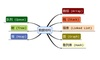数据结构 订阅